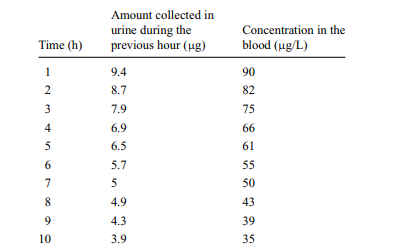# In the previous problem, is the value of V that you determined equal to the volume of the person?

In the previous problem, is the value of V that you determined equal to the volume of the person? Does this make sense to you? If not, what does V represent?

Previous problem

A person consumes 100 µg of a tracer chemical. Assume that the person is able to collect all of the tracer in their urine (and therefore measure the amount that has come out of the body), as well as the concentration in the blood, as a function of time (see table).

a. Is a first-order rate constant appropriate for describing the process of elimination via the kidneys? Justify your answer.

b. Assuming that the answer to a is “yes,” find the rate constant k and the total volume V from these data.